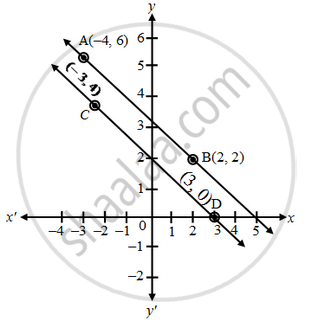# Show graphically that the system of equations 2x + 3y = 10, 4x + 6y = 12 has no solution - Mathematics

Sum

Show graphically that the system of equations 2x + 3y = 10, 4x + 6y = 12 has no solution

#### Solution

The given equations are

2x + 3y = 10 ⇒ y = \frac { 10-2x }{ 3 }

 x -4 2 y 6 2 Points A B

4x + 6y = 12     ⇒      y =   \frac { 12-4x }{ 6 }

 x -3 3 y 4 0 Points C D

Plot the points A (–4, 6), B(2, 2) and join them to form a line AB. Similarly, plot the points C(–3, 4), D(3, 0) and join them to get a line CDClearly, the graphs of the given equations are parallel lines. As they have no common point, there is no common solution. Hence, the given system of equations has no solution.

Concept: Graphical Method of Solution of a Pair of Linear Equations
Is there an error in this question or solution?